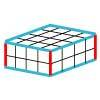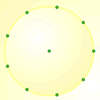#### You may also like### Round and Round the Circle

What happens if you join every second point on this circle? How about every third point? Try with different steps and see if you can predict what will happen.### Making Cuboids

Let's say you can only use two different lengths - 2 units and 4 units. Using just these 2 lengths as the edges how many different cuboids can you make?How many DIFFERENT quadrilaterals can be made by joining the dots on the 8-point circle?

# Exploring Number Patterns You Make

##### Age 7 to 11 Challenge Level:
Seth from Middleton Tyas C of E Primary School explored the rule 'times by 9 and then subtract 7' and the starting number used was 8:

Number Patterns
[Rule: x9 -7]

 8 65 578 5195 46748 420725 3786518 34078655

Having completed eight calculations,I have noticed that 8 and 5 are recurring digits in the units column, in this order: 8, 5, 8, 5, 8, 5 etc, from even to odd.
I have also noticed that the third and fourth, fifth and sixth, seventh and eighth numbers are pairs and decrease in order.

Bobby, Kaiden and Ella from Holy Trinity Primary School in Halstead sent in the following:

We discovered that every number which was timesed by four and then had five deducted from it was always an odd number. For example: Our number was zero, we then timesed it by four which left us with zero, next we deducted five and our final answer was minus five.
We did this to find out how these two operations change a number in different ways. We also did this because it will help our understanding of the operations times and divide, this will also help us to learn how to do more than one number sentence to get an answer. Doing this will also increase our understanding of maths and our maths skills.

Jamie from St. Ebbes Primary School in Oxford wrote:

I think that if you do the solution, the unit ends in 3 or 7,

eg: 3, 7, 23, 87, 343, 1367, 5463.

Also the number that has been multiplied by 4 you take away 5.

Yes your last line is the rule that was used in the example, and you noticed the repeating 3, 7 - I wonder if anyone knows why this happens.

Thank you for sharing what you explored.  There were some other solutions that came in that stuck to the example shown and saw the repeating pattern in the units.